##SYSTEMS OF EQUATIONS in TWO VARIABLESA system of equations is a collection of two or more equations with the same set of unknowns. In solving a system of equations, we try to find values for each of the unknowns that will satisfy every equation in the system.

The equations in the system can be linear or non-linear. This tutorial reviews systems of linear equations.

A problem can be expressed in narrative form or the problem can be expressed in algebraic form.

Let's start with an example stated in narrative form. We'll convert it to an equivalent equation in algebraic form, and then we will solve it.

Example 1:

A total of $12,000 is invested in two funds paying 9% and 11% simple interest. If the yearly interest is$1,180, how much of the $12,000 is invested at each rate? Before you work this problem, you must know the definition of simple interest. Simple interest can be calculated by multiplying the amount invested at the interest rate. Solution: We have two unknowns: the amount of money invested at 9% and the amount of money invested at 11%. Our objective is to find these two numbers. Sentence (1) ''A total of$12,000 is invested in two funds paying 9% and 11% simple interest.'' can be restated as (The amount of money invested at 9%)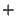(The amount of money invested at 11%)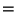$12,000. Sentence (2) ''If the yearly interest is$1,180, how much of the $12,000 is invested at each rate?'' can be restated as (The amount of money invested at 9%)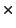9% + (The amount of money invested at 11%11%)total interest of$1,180.

It is going to get tiresome writing the two phrases (The amount of money invested at 9%) and (The amount of money invested at 11%) over and over again. So let's write them in shortcut form. Call the phrase (The amount of money invested at 9%) by the symbol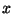and call the phrase (The amount of money invested at 11%) by the symbol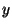.

Let's rewrite sentences (1) and (2) in shortcut form.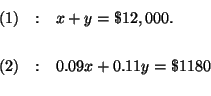We have converted a narrative statement of the problem to an equivalent algebraic statement of the problem. Let's solve this system of equations.

A system of linear equations can be solved four different ways:

Substitution,

Elimination,

Matrices,

Graphing.

The Method of Substitution:

The method of substitution involves five steps:

Step 1: Solve for y in equation (1).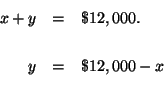Step 2: Substitute this value for y in equation (2). This will change equation (2) to an equation with just one variable, x.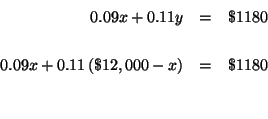Step 3: Solve for x in the translated equation (2).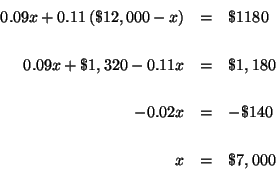Step 4: Substitute this value of x in the y equation you obtained in Step 1.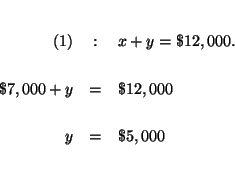Step 5: Check your answers by substituting the values of x and y in each of the original equations. If, after the substitution, the left side of the equation equals the right side of the equation, you know that your answers are correct.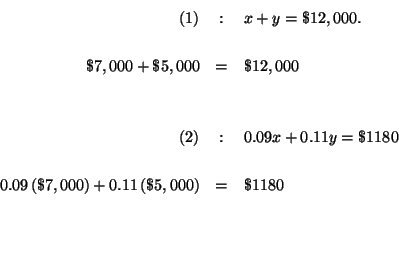The Method of Elimination:

The process of elimination involves five steps:

In a two-variable problem rewrite the equations so that when the equations are added, one of the variables is eliminated, and then solve for the remaining variable.

Step 1: Change equation (1) by multiplying equation (1) by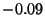to obtain a new and equivalent equation (1).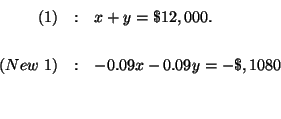Step 2: Add new equation (1) to equation (2) to obtain equation (3).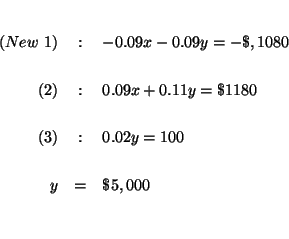Step 3: Substitute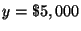in equation (1) and solve for x.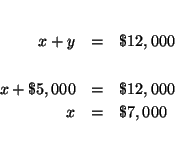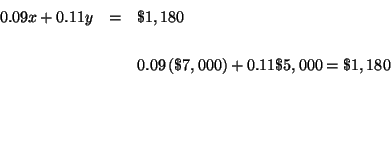The Method of Matrices:

This method is essentially a shortcut for the method of elimination.

Rewrite equations (1) and (2) without the variables and operators. The left column contains the coefficients of the x's, the middle column contains the coefficients of the y's, and the right column contains the constants.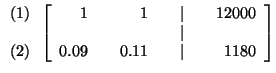The objective is to reorganize the original matrix into one that looks like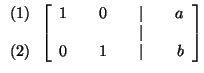where a and b are the solutions to the system.

Step 1. Manipulate the matrix so that the number in cell 11 (row 1-col 1) is 1. In this case, we don't have to do anything. The number 1 is already in the cell.

Step 2: Manipulate the matrix so that the number in cell 21 is 0. To do this we rewrite the matrix by keeping row 1 and creating a new row 2 by adding -0.09 x row 1 to row 2.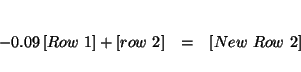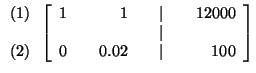Step 3: Manipulate the matrix so that the cell 22 is 1. Do this by multiplying row 2 by 50.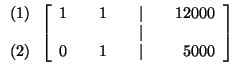Step 4: Manipulate the matrix so that cell 12 is 0. Do this by adding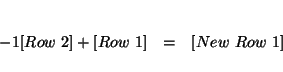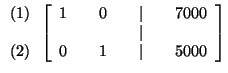You can read the answers off the matrix as x = $7,000 and y =$5,000.

The method of Graphing:

In this method solve for y in each equation and graph both. The point of intersection is the solution.

If you would like to work a similar example, click on Example.

If you would like to test yourself by working some problem similar to this example, click on Problem.

This site was built to accommodate the needs of students. The topics and problems are what students ask for. We ask students to help in the editing so that future viewers will access a cleaner site. If you feel that some of the material in this section is ambiguous or needs more clarification, or if you find a mistake, please let us know by e-mail at sosmath.com.[Next Example] [Next Problem]
[Three-Variable Systems]
[Algebra] [Geometry] [Trigonometry ]S.O.S MATH: Home Page

Do you need more help? Please post your question on our S.O.S. Mathematics CyberBoard.Author: Nancy Marcus